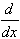#Interactive Real Analysis

Next | Previous | Glossary | Map

## 7.2. Integration Techniques

### Theorem 7.2.5: Integration by Parts

Suppose f and g are two continuously differentiable functions. Let G(x) = f(x) g(x). Thenf(x) g'(x) dx = ( G(b) - G(a) ) -f'(x) g(x) dx

### Proof:

For the function G(x) = f(x) g(x) we have by the Product Rule:G(x) =[ f(x) g(x) ] = f'(x) g(x) + f(x) g'(x)
Therefore the function G is an antiderivative of the function f'(x) g(x) + f(x) g'(x) which means that
G(b) - G(a) =f'(x) g(x) + f(x) g'(x) dx =
=f'(x) g(x) dx +f(x) g'(x) dx
But that is equivalent to the statement we want to prove.
Next | Previous | Glossary | Map